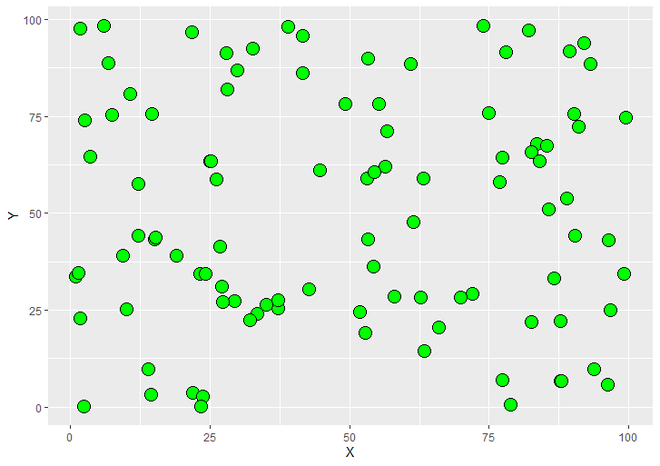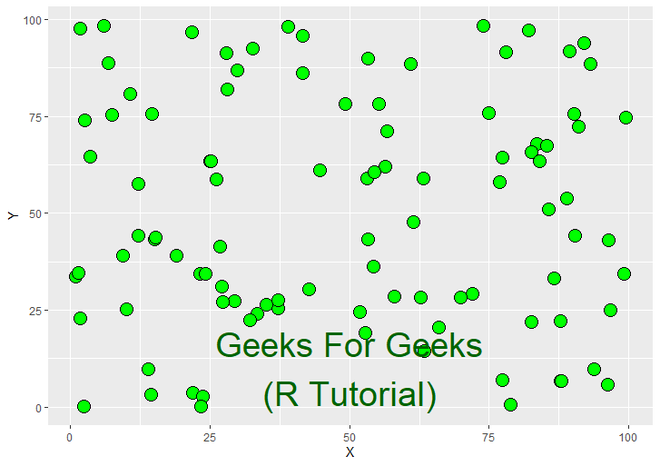# Annotate Multiple Lines of Text to ggplot2 Plot in R

• Last Updated : 07 Jul, 2022

In this article, we will see how to annotate Multiple Lines of text to ggplot2 Plot in R programming language.

Let us first create a regular plot so that the difference is apparent,

Example:

## R

 `# Load Package``library``(``"ggplot2"``)` `# Create a DataFrame``DF <- ``data.frame``(X = ``runif``(100, min=0, max=100),                       ``                 ``Y = ``runif``(100, min=0, max=100))` `# Create a ScatterPlot using ggplot2.``ggplot``(DF,``aes``(X, Y))+``  ``geom_point``(size = 5, fill = ``"green"``, color = ``"black"``, shape = 21)`

Output:ScatterPlot using ggplot2

Now to annotate multiple lines to the plot annotate() function is used along the regular plot. This function is passed the required value and attributes. The approach is similar to adding a single line to the plot, only difference being each new line should be created by using “\n” within the text that is to be passed to the plot.

Syntax : annotate(geom, x, y, label)

Parameters :

• geom : We assign geom that we want to add to the plot as a first parameter which will written in “” . geom can be anything like rect, segment, pointrange etc. here we will use text as a geom as we want to add text. all other arguments will be depends on geom that we select. So here all other parameters that we will use are only works for Annotating text to plot.
• x : Represents the Co-ordinates of X Axis.
• y : Represents the Co-ordinates of Y Axis.
• label : Text that we want to annotate on plot.
• Above threes parameters (i.e x, y and label) are necessary for annotating text but here we will also use two parameters size and color which are used to represent size and color of text respectively and they are not necessary to use.

Return : Geoms on plot.

Example:

## R

 `# Load ggplot2 Package``library``(``"ggplot2"``)` `# Create a DataFrame for Plotting``DF <- ``data.frame``(X = ``runif``(100, min=0, max=100),                       ``                 ``Y = ``runif``(100, min=0, max=100))` `# ScatterPlot with Multiple Lines Text on it.``ggplot``(DF,``aes``(X, Y))+``  ``geom_point``(size = 5, fill = ``"green"``, color = ``"black"``, shape = 21)+``  ``annotate``(``"text"``, x = 50, y = 10, label = ``"Geeks For Geeks\n(R Tutorial)"``,``           ``size = 10, color = ``"dark green"``)`

Output:ScatterPlot with Annotating Multiple Lines on Plot

My Personal Notes arrow_drop_up